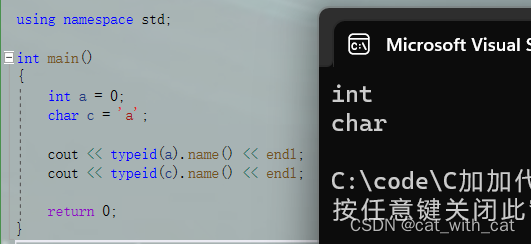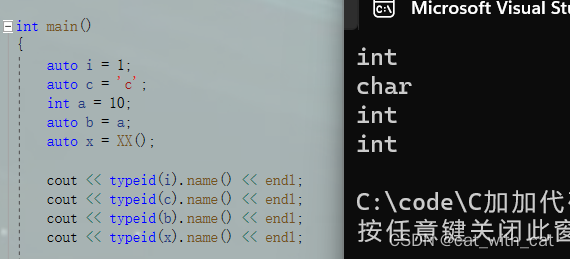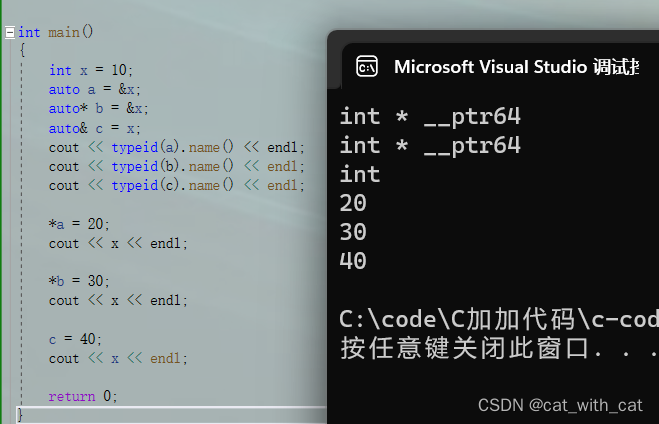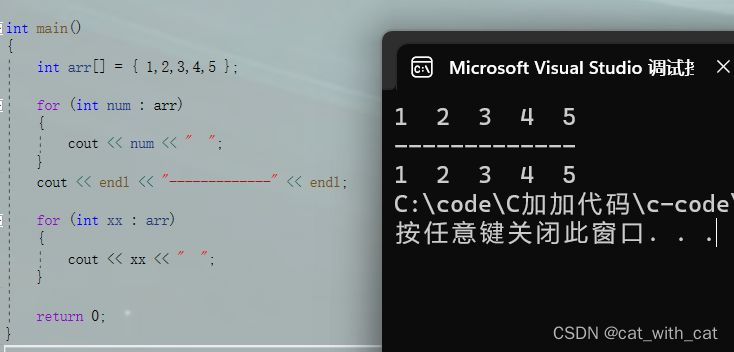# auto关键字

## 定义

C++11中，标准委员会赋予了auto全新的含义即：auto不再是一个存储类型指示符，而是作为一个新的类型指示符来指示编译器，auto声明的变量必须由编译器在编译时期推导而得

## 补充知识点：typeid

``````#include<iostream>
#include<typeinfo>

using namespace std;

int main()
{
int a = 0;
char c = 'a';

cout << typeid(a).name() << endl;
cout << typeid(c).name() << endl;

return 0;
}
````````````#include<iostream>
#include<typeinfo>

using namespace std;

int XX()
{
return 1;
}

int main()
{
auto i = 1;
auto c = 'c';
int a = 10;
auto b = a;
auto x = XX();

cout << typeid(i).name() << endl;
cout << typeid(c).name() << endl;
cout << typeid(b).name() << endl;
cout << typeid(x).name() << endl;

return 0;
}
``````## 详细规则

### 变量初始化

1.使用auto时，必须对其修饰的变量进行初始化，不然编译器无法得知该变量是什么类型，会报错

### auto与指针和引用

2.auto和指针以及引用结合使用

auto和指针结合使用的时候，*可写可不写

auto和引用结合使用的时候，&必须写

``````#include<iostream>
#include<typeinfo>

using namespace std;

int main()
{
int x = 10;
auto a = &x;
auto* b = &x;
auto& c = x;
cout << typeid(a).name() << endl;
cout << typeid(b).name() << endl;
cout << typeid(c).name() << endl;

*a = 20;
cout << x << endl;

*b = 30;
cout << x << endl;

c = 40;
cout << x << endl;

return 0;
}
``````### 一次定义多个变量

``````#include<iostream>

using namespace std;

int main()
{
auto a = 1, b = 2, c = 3;

return 0;
}
``````

### auto不能做的

auto定义的变量不能作为函数的参数

auto不能直接定义数组

# 范围内的for循环

## 语法格式

``````for (element_type variable : container)
{

}
``````

element_type是要便利的容器内元素的类型
variable 是变量名称，类似于给容器内单个元素进行命名
container是要遍历的容器的名称

``````#include<iostream>

using namespace std;

int main()
{
int arr[] = { 1,2,3,4,5 };

for (int num : arr)
{
cout << num << "  ";
}
cout << endl << "-------------" << endl;

for (int xx : arr)
{
cout << xx << "  ";
}

return 0;
}
``````